# Zoom, Seniors, What’s Next, How to Email An Admission Counselor# Hello, The College Map!

## The office will be closed on Labor Day. Previously scheduled test prep will still be held.

 table div table+table+table+table+table div table{width:100%;padding:0}table div table+table+table+table+table div table img{width:96.23%;padding:0;float:none}table div table+table+table+table+table div table td{width:100%;padding:0 1.88% 18px}/* styles */## Virtual College Tours & Fair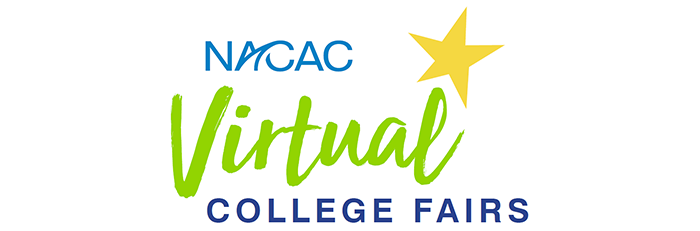/* styles */ NACAC Virtual College Fair Here's your chance to hear firsthand from those-in-the-know at hundreds of colleges and universities. How? Attend a NACAC Virtual College Fair! Dates: 9/13, 10/12, 10/18 and 11/8. To view the fall schedule and to sign up, click HERE. Parents are also welcome to attend.
 table div table+table+table+table+table+table+table+table+table div table{width:100%;padding:0}table div table+table+table+table+table+table+table+table+table div table img{width:96.23%;padding:0;float:none}table div table+table+table+table+table+table+table+table+table div table td{width:100%;padding:0 1.88% 18px}/* styles */Below is a great link to over 900 colleges/universities with links to virtual tours and unique features of each school all in one place. This is a great one-stop shop for our students that are unable to make in-person visits.

 table div table+table+table+table+table+table+table+table+table+table+table div table{width:100%;padding:0}table div table+table+table+table+table+table+table+table+table+table+table div table img{width:96.23%;padding:0;float:none}table div table+table+table+table+table+table+table+table+table+table+table div table td{width:100%;padding:0 1.88% 18px}/* styles */Several college planning parents have reach out and asked for us to share the introduction from the zoom senior parent meeting on 8/29/20. We were experiencing some technical difficulties. A special thanks to all who were in attendance!

Good morning, welcome, and thank you for joining us this morning. We are honored to work with your sons and daughters. As we enter this application season, we are keenly aware that the current climate and culture of COVID-19 has many of our students and families concerned about the impact on admission decisions. And while we acknowledge there are some uncertainties unique to this admission cycle, the core of our services, the industry, and the end product has not wavered. We lean on our team members' individual and collective years of experience to navigate this landscape and continue to guide your student well. We ask for your continued trust and faith in our partnership.

 table div table+table+table+table+table+table+table+table+table+table+table+table+table div table{width:100%;padding:0}table div table+table+table+table+table+table+table+table+table+table+table+table+table div table img{width:96.23%;padding:0;float:none}table div table+table+table+table+table+table+table+table+table+table+table+table+table div table td{width:100%;padding:0 1.88% 18px}/* styles */## September RemindersClass of 2021 September Reminders:

Seniors, set up a meeting with your guidance counselor to review your updated transcripts with accurate gpa, class size and rank. Make sure all grade and courses listed are accurate, including any high school credits earned through middle and virtual school.

Many colleges require "recommenders". Confirm the colleges on your list that require letters of recommendation. Email and kindly ask your guidance counselor and two teachers if they are willing to write you a letter of recommendation. Attach your resume. Please respect their time. Make sure to give them a minimum of a three week notice.

▪ Make sure all of your Common Application essay drafts are finalized and ready to go.
▪ Consider applying Early Decision and/or Early Action.
▪ Begin working on supplemental essays for schools that require them. Make sure to create your google doc and share it with your essay editor.

Class of 2022 Reminders:

▪ Continue with Test Prep.
▪ Begin work on resumes and college lists
▪ Continue with virtual college visits!
 ▪ Continue with Test Prep.
 ▪ Begin work on resumes and college lists
 ▪ Continue with virtual college visits!
 table div table+table+table+table+table+table+table+table+table+table+table+table+table+table+table+table div table{width:100%;padding:0}table div table+table+table+table+table+table+table+table+table+table+table+table+table+table+table+table div table img{width:96.23%;padding:0;float:none}table div table+table+table+table+table+table+table+table+table+table+table+table+table+table+table+table div table td{width:100%;padding:0 1.88% 18px}/* styles */## Seniors, what's next?

Class of 2021

Boot Camp is over, what next? Ready, Set, Apply!

▪ Continue to meet one-on-one with your college counselor
▪ Final edits of your Common App Essay if you are still working on it
▪ Final edits to your Resume if that has not been completed
▪ Begin opening and working within each of your college applications. Review the requirements and if a supplemental essay is required, please create a google doc and list the supplements by school name and due date. Make sure to share this new google doc with your essay editor.
 ▪ Continue to meet one-on-one with your college counselor
 ▪ Final edits of your Common App Essay if you are still working on it
 ▪ Final edits to your Resume if that has not been completed
 ▪ Begin opening and working within each of your college applications. Review the requirements and if a supplemental essay is required, please create a google doc and list the supplements by school name and due date. Make sure to share this new google doc with your essay editor.

Remember all of our applications deadlines are one month prior to the schools posted deadline.

Please make sure a TCM counselor reviews your applications before you submit.

 table div table+table+table+table+table+table+table+table+table+table+table+table+table+table+table+table+table+table+table div table{width:100%;padding:0}table div table+table+table+table+table+table+table+table+table+table+table+table+table+table+table+table+table+table+table div table img{width:96.23%;padding:0;float:none}table div table+table+table+table+table+table+table+table+table+table+table+table+table+table+table+table+table+table+table div table td{width:100%;padding:0 1.88% 18px}/* styles */## What is the FAFSA?The FAFSA (Free Application for Federal Student Aid) opens on October 1, 2020.

Every student and (1) parent will need to create an FSA ID. The FSA ID allows students and parents to identify themselves electronically to access Federal Student Aid websites. An FSA ID is made up of a username and password and can be used to log into the online FAFSA form. Click here for a quick video.

Most all colleges and universities require a FAFSA to be filed in order to be eligible for institutional scholarships.

Here is a great website with useful tips, checklists and many resources.

 table div table+table+table+table+table+table+table+table+table+table+table+table+table+table+table+table+table+table+table+table+table+table div table{width:100%;padding:0}table div table+table+table+table+table+table+table+table+table+table+table+table+table+table+table+table+table+table+table+table+table+table div table img{width:96.23%;padding:0;float:none}table div table+table+table+table+table+table+table+table+table+table+table+table+table+table+table+table+table+table+table+table+table+table div table td{width:100%;padding:0 1.88% 18px}/* styles */## How to Email an Admissions Counselortable div table+table+table+table+table+table+table+table+table+table+table+table+table+table+table+table+table+table+table+table+table+table+table+table+table+table div table{width:100%;padding:0}table div table+table+table+table+table+table+table+table+table+table+table+table+table+table+table+table+table+table+table+table+table+table+table+table+table+table div table img{width:96.23%;padding:0;float:none}table div table+table+table+table+table+table+table+table+table+table+table+table+table+table+table+table+table+table+table+table+table+table+table+table+table+table div table td{width:100%;padding:0 1.88% 18px}/* styles */table div table+table+table+table+table+table+table+table+table+table+table+table+table+table+table+table+table+table+table+table+table+table+table+table+table+table+table+table div table,table.module-27{width:32.08%;float:left;padding:0}table div table+table+table+table+table+table+table+table+table+table+table+table+table+table+table+table+table+table+table+table+table+table+table+table+table+table+table+table div table a{border:0 none;text-decoration:none}table div table+table+table+table+table+table+table+table+table+table+table+table+table+table+table+table+table+table+table+table+table+table+table+table+table+table+table+table div table img{width:100%!important;border:0 none;text-decoration:none}table div table+table+table+table+table+table+table+table+table+table+table+table+table+table+table+table+table+table+table+table+table+table+table+table+table+table+table+table div table td{width:100%;padding:0 20px 20px 0}/* styles */ What is Bright Futures? It opens on December 1st?
 table div table+table+table+table+table+table+table+table+table+table+table+table+table+table+table+table+table+table+table+table+table+table+table+table+table+table+table+table+table div table,table.module-28{width:32.08%;float:left;padding:0}table div table+table+table+table+table+table+table+table+table+table+table+table+table+table+table+table+table+table+table+table+table+table+table+table+table+table+table+table+table div table a{border:0 none;text-decoration:none}table div table+table+table+table+table+table+table+table+table+table+table+table+table+table+table+table+table+table+table+table+table+table+table+table+table+table+table+table+table div table img{width:100%!important;border:0 none;text-decoration:none}table div table+table+table+table+table+table+table+table+table+table+table+table+table+table+table+table+table+table+table+table+table+table+table+table+table+table+table+table+table div table td{width:100%;padding:0 20px 20px 0}/* styles */ How do I register for the October 3rd SAT Subject Test?
 table div table+table+table+table+table+table+table+table+table+table+table+table+table+table+table+table+table+table+table+table+table+table+table+table+table+table+table+table+table+table div table,table.module-29{width:32.08%;float:left;padding:0}table div table+table+table+table+table+table+table+table+table+table+table+table+table+table+table+table+table+table+table+table+table+table+table+table+table+table+table+table+table+table div table a{border:0 none;text-decoration:none}table div table+table+table+table+table+table+table+table+table+table+table+table+table+table+table+table+table+table+table+table+table+table+table+table+table+table+table+table+table+table div table img{width:100%!important;border:0 none;text-decoration:none}table div table+table+table+table+table+table+table+table+table+table+table+table+table+table+table+table+table+table+table+table+table+table+table+table+table+table+table+table+table+table div table td{width:100%;padding:0 20px 20px 0}/* styles */ Test-Optional Colleges: what does that mean?
 table div table+table+table+table+table+table+table+table+table+table+table+table+table+table+table+table+table+table+table+table+table+table+table+table+table+table+table+table+table+table+table div table,table.module-30{width:32.08%;float:left;padding:0}table div table+table+table+table+table+table+table+table+table+table+table+table+table+table+table+table+table+table+table+table+table+table+table+table+table+table+table+table+table+table+table div table a{border:0 none;text-decoration:none}table div table+table+table+table+table+table+table+table+table+table+table+table+table+table+table+table+table+table+table+table+table+table+table+table+table+table+table+table+table+table+table div table img{width:100%!important;border:0 none;text-decoration:none}table div table+table+table+table+table+table+table+table+table+table+table+table+table+table+table+table+table+table+table+table+table+table+table+table+table+table+table+table+table+table+table div table td{width:100%;padding:0 20px 20px 0}/* styles */ Do I need To Take an SAT Subject Tests?
 table div table+table+table+table+table+table+table+table+table+table+table+table+table+table+table+table+table+table+table+table+table+table+table+table+table+table+table+table+table+table+table+table div table{width:100%;padding:0}table div table+table+table+table+table+table+table+table+table+table+table+table+table+table+table+table+table+table+table+table+table+table+table+table+table+table+table+table+table+table+table+table div table img{width:96.23%;padding:0;float:none}table div table+table+table+table+table+table+table+table+table+table+table+table+table+table+table+table+table+table+table+table+table+table+table+table+table+table+table+table+table+table+table+table div table td{width:100%;padding:0 1.88% 18px}/* styles */## Senior Transcript Orders!/* styles */ Seniors in our College Planning program: Your junior year grades should officially be finalized and processed on your transcript by mid June. Please order an unofficial copy from your school. We need to verify the transcript for accuracy over the summer to give a head-start on any possible corrections that need to be made. Remember: you'll be sending official transcripts to each college you apply to; it's imperative that they be accurate and updated prior to that time. Note: We will check it one final time before you send the official copies to colleges (that particular version must have your senior year schedule on it, which usually happens in September of your senior year).
 table div table+table+table+table+table+table+table+table+table+table+table+table+table+table+table+table+table+table+table+table+table+table+table+table+table+table+table+table+table+table+table+table+table+table+table+table div table{width:100%;padding:0}table div table+table+table+table+table+table+table+table+table+table+table+table+table+table+table+table+table+table+table+table+table+table+table+table+table+table+table+table+table+table+table+table+table+table+table+table div table img{width:96.23%;padding:0;float:none}table div table+table+table+table+table+table+table+table+table+table+table+table+table+table+table+table+table+table+table+table+table+table+table+table+table+table+table+table+table+table+table+table+table+table+table+table div table td{width:100%;padding:0 1.88% 18px}/* styles */## Should I Declare a Major on My College Applications?table div table+table+table+table+table+table+table+table+table+table+table+table+table+table+table+table+table+table+table+table+table+table+table+table+table+table+table+table+table+table+table+table+table+table+table+table+table+table+table+table div table{width:100%;padding:0}table div table+table+table+table+table+table+table+table+table+table+table+table+table+table+table+table+table+table+table+table+table+table+table+table+table+table+table+table+table+table+table+table+table+table+table+table+table+table+table+table div table img{width:96.23%;padding:0;float:none}table div table+table+table+table+table+table+table+table+table+table+table+table+table+table+table+table+table+table+table+table+table+table+table+table+table+table+table+table+table+table+table+table+table+table+table+table+table+table+table+table div table td{width:100%;padding:0 1.88% 18px}/* styles */table div table+table+table+table+table+table+table+table+table+table+table+table+table+table+table+table+table+table+table+table+table+table+table+table+table+table+table+table+table+table+table+table+table+table+table+table+table+table+table+table+table+table+table div table{width:100%;padding:0}table div table+table+table+table+table+table+table+table+table+table+table+table+table+table+table+table+table+table+table+table+table+table+table+table+table+table+table+table+table+table+table+table+table+table+table+table+table+table+table+table+table+table+table div table img{width:96.23%;padding:0;float:none}table div table+table+table+table+table+table+table+table+table+table+table+table+table+table+table+table+table+table+table+table+table+table+table+table+table+table+table+table+table+table+table+table+table+table+table+table+table+table+table+table+table+table+table div table td{width:100%;padding:0 1.88% 18px}/* styles */## 2020-2021 TEST PREP OVERVIEW

Dear Test Prep Families,

Welcome to a new test prep cycle at The College Map! We look forward to a very purposeful and productive shared partnership with you from now until the June 2021 ACT or SAT test dates. Here is a summary email of everything you will need to know this year:

The College Map’s Test Prep Philosophy:
Simply stated, we believe in the gift of time: repetition, progression, and mastery over time. Both the SAT and ACT are formulaic, predictable exams. Skills and strategies can be taught to the test and reinforced. We expose our students to timed simulated testing with immediate feedback and instruction, which includes skill-based teaching as well as strategy-based teaching. We do this through live instruction as well as an online practice test delivery mechanism.

Live Prep Sessions:
We offer SAT and ACT verbal and math classes taught in two-hour blocks. We use a “three S” approach to teaching these classes: Skills, Strategy, and Simulation. The fall class schedule is posted to our password-protected page, “Your Path,” on our website (www.TheCollegeMap.com). The password is prepare@TCM2020. It is case-sensitive.
Each student has his/her own notebook housed at The College Map that holds formula/reference sheets specific to each subject of the exam, class notes, and sample test problems completed in class.

Online Prep Sessions:
Students have an online test prep account (www.eprep.com) that houses their practice tests. Students are expected to complete one full-length practice test each nine week grading period. Each time a student comes to The College Map to work on practice tests, they will complete a single section of one practice test. We kindly ask that students bring their own calculators and headphones to each online prep session.

The ACT has four sections: English, Math, Reading, and Science. The SAT has four sections: Writing, Reading, Math No Calculator, and Math with Calculator. Each session or section of the test is designed to be completed within a 2-hour time frame. Students take a paper practice test section simulating test conditions: no phones, quiet atmosphere, timer utilized, answer sheet used, etc. Once a student completes the paper/pencil section of the test, they input answers into a software program. The program grades the test, provides feedback on questions correct or incorrect and provides a score similar to the score the student would actually earn on the SAT or ACT. The student then immediately watches instructional videos for any guessed-at or incorrect questions.
Students who view the video lesson tutorials on each question they missed on the practice tests have greater score increases on actual tests. This step is critical to test mastery!Having students on-site for the completion of practice tests provides multiple benefits: allows instructors to establish relationships with the student
allows instructors to learn the student’s problem areas and tailor the live instruction to teach on those areas eliminates the need for the parent to monitor the student’s progress from home.

1. ePrep:
As mentioned above, this is a student’s practice account. It can be accessed either directly through the ePrep website or through our “Your Path” password-protected page. A student’s username is his/her email address and the password is the student’s first name, then “TCM,” and graduation year. All lowercase. For example: julietcm2020. Parents, we strongly encourage you to have your son/daughter log into their ePrep account to show you how it works, but most importantly, to view his/her progress.
2. Acuity:
This is the online scheduling vehicle (think “Sign Up Genius”) that we use for live prep session and online practice session scheduling for our students. Acuity can be accessed either through their website
(www.timetocollegemap.acuityscheduling.com) or through “Your Path” on The College Map’s website homepage. Students should use the online and live prep calendars posted on “Your Path” and select corresponding dates in Acuity to reserve their class spots (we cap class sizes in order to provide individualized instructional attention). Once in Acuity, students will create an account. You must be registered through Acuity to attend any type of class, mock exam, etc.
3. Cancellation Policy:
Students are responsible for keeping track of their prep sessions, showing up, and completing the work designated. Students must cancel or reschedule classes before 24-hours preceding the class start time to avoid a cancellation fee. Students are allowed one missed ("NO SHOW") online and/or live test prep session (non-canceled) without penalty. A student will always receive an email acknowledging the absence and reminding them about our cancellation policy. Cancellation fees are assessed in this manner:
\$50 for the second missed class;
\$65 for the third;
\$85 for the fourth.
Parents, in our experience, it is most effective to have the student pay this fee. Our goal is to cultivate an understanding of the importance of test prep and a habit of responsibility and accountability. Two things occur when a student misses a class: his/her test score is not maximized, and another student missed an opportunity to be in class. Classes canceled 24 hours prior to the appointment time should be canceled through Acuity. The only excused reason for a class being canceled less than 24 hours in advance is for illness. In this event, please email me at juliemitchell@thecollegemap.com.
4. Formula for Success:
Students should attend a minimum of four live prep math and verbal sessions before each real test the student plans to take. We anticipate that our students will take two fall and two springs real tests. Students who follow this formula for success should expect to see a 7 point ACT gain or a 240 point SAT gain from their diagnostic test score.
The goal is for students to take two fall and two spring tests.
Consider your student’s academic workload and extracurricular schedule. Students have very full and busy lives. Your student will need to schedule eight two-hour test prep sessions leading up to each test. You want to consider how you will schedule these two-hour prep sessions into their already busy schedule.
6. Registering for the SAT or ACT:
Please register for the ACT at act.org and SAT at collegeboard.org. Please note the following early registration deadline dates (you can register after the deadline date, but a late fee will be required). We recommend registering at least once for the “Writing” (essay) portion of the ACT or SAT although many colleges will not consider it in their admission decisions nor are requiring it.
7. Reporting Your Scores to Us:
On the first day that SAT or ACT specific exams date scores are scheduled to be released, I will email students to log into either their SAT or ACT accounts to check. It is very important that students email me their results for every test attempted as I compile ongoing student data. A student’s data assists our test prep instruction team in evaluating our live prep session content as well as in tweaking a particular student’s test prep program.
8. Bright Futures:
This is the lottery funded state of Florida scholarship opportunity for high school students. ACT and SAT can both be super scored to qualify (super scoring is taking the highest verbal and math score from any test administration date and combining them to achieve the highest composite score possible.) To qualify for the Florida Academic Scholarship, students will need a minimum of a 29 on the ACT or a 1330 on the SAT. To qualify for the Florida Medallion Scholarship, students will need a minimum of a 25 on the ACT or a 1210 on the SAT.
 1 ePrep: As mentioned above, this is a student’s practice account. It can be accessed either directly through the ePrep website or through our “Your Path” password-protected page. A student’s username is his/her email address and the password is the student’s first name, then “TCM,” and graduation year. All lowercase. For example: julietcm2020. Parents, we strongly encourage you to have your son/daughter log into their ePrep account to show you how it works, but most importantly, to view his/her progress.
 2 Acuity: This is the online scheduling vehicle (think “Sign Up Genius”) that we use for live prep session and online practice session scheduling for our students. Acuity can be accessed either through their website (www.timetocollegemap.acuityscheduling.com) or through “Your Path” on The College Map’s website homepage. Students should use the online and live prep calendars posted on “Your Path” and select corresponding dates in Acuity to reserve their class spots (we cap class sizes in order to provide individualized instructional attention). Once in Acuity, students will create an account. You must be registered through Acuity to attend any type of class, mock exam, etc.
 3 Cancellation Policy: Students are responsible for keeping track of their prep sessions, showing up, and completing the work designated. Students must cancel or reschedule classes before 24-hours preceding the class start time to avoid a cancellation fee. Students are allowed one missed ("NO SHOW") online and/or live test prep session (non-canceled) without penalty. A student will always receive an email acknowledging the absence and reminding them about our cancellation policy. Cancellation fees are assessed in this manner: \$50 for the second missed class; \$65 for the third; \$85 for the fourth. Parents, in our experience, it is most effective to have the student pay this fee. Our goal is to cultivate an understanding of the importance of test prep and a habit of responsibility and accountability. Two things occur when a student misses a class: his/her test score is not maximized, and another student missed an opportunity to be in class. Classes canceled 24 hours prior to the appointment time should be canceled through Acuity. The only excused reason for a class being canceled less than 24 hours in advance is for illness. In this event, please email me at juliemitchell@thecollegemap.com.
 4 Formula for Success: Students should attend a minimum of four live prep math and verbal sessions before each real test the student plans to take. We anticipate that our students will take two fall and two springs real tests. Students who follow this formula for success should expect to see a 7 point ACT gain or a 240 point SAT gain from their diagnostic test score.
 5 Choosing Your Test Date: The goal is for students to take two fall and two spring tests. Consider your student’s academic workload and extracurricular schedule. Students have very full and busy lives. Your student will need to schedule eight two-hour test prep sessions leading up to each test. You want to consider how you will schedule these two-hour prep sessions into their already busy schedule.
 6 Registering for the SAT or ACT: Please register for the ACT at act.org and SAT at collegeboard.org. Please note the following early registration deadline dates (you can register after the deadline date, but a late fee will be required). We recommend registering at least once for the “Writing” (essay) portion of the ACT or SAT although many colleges will not consider it in their admission decisions nor are requiring it.
 7 Reporting Your Scores to Us: On the first day that SAT or ACT specific exams date scores are scheduled to be released, I will email students to log into either their SAT or ACT accounts to check. It is very important that students email me their results for every test attempted as I compile ongoing student data. A student’s data assists our test prep instruction team in evaluating our live prep session content as well as in tweaking a particular student’s test prep program.
 8 Bright Futures: This is the lottery funded state of Florida scholarship opportunity for high school students. ACT and SAT can both be super scored to qualify (super scoring is taking the highest verbal and math score from any test administration date and combining them to achieve the highest composite score possible.) To qualify for the Florida Academic Scholarship, students will need a minimum of a 29 on the ACT or a 1330 on the SAT. To qualify for the Florida Medallion Scholarship, students will need a minimum of a 25 on the ACT or a 1210 on the SAT.

We value our partnership with you in making your student as successful as possible in our test prep program. Please reach out to me any time you have a question.
Warmly,
Julie Mitchell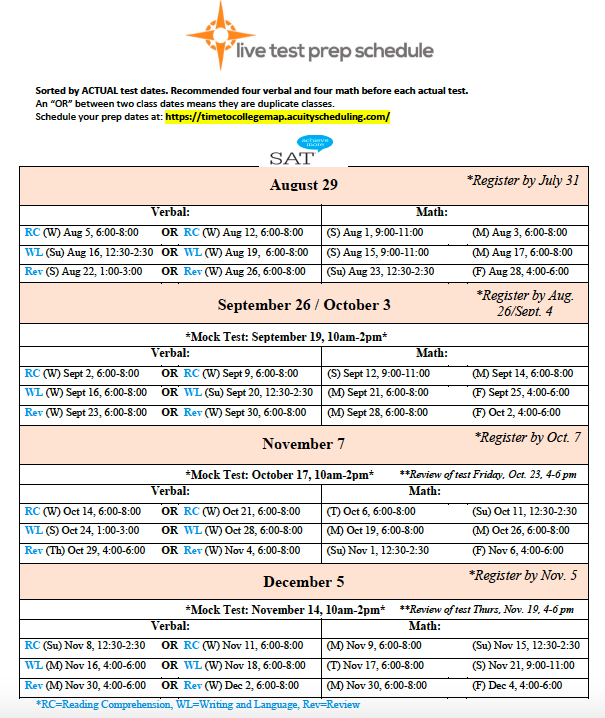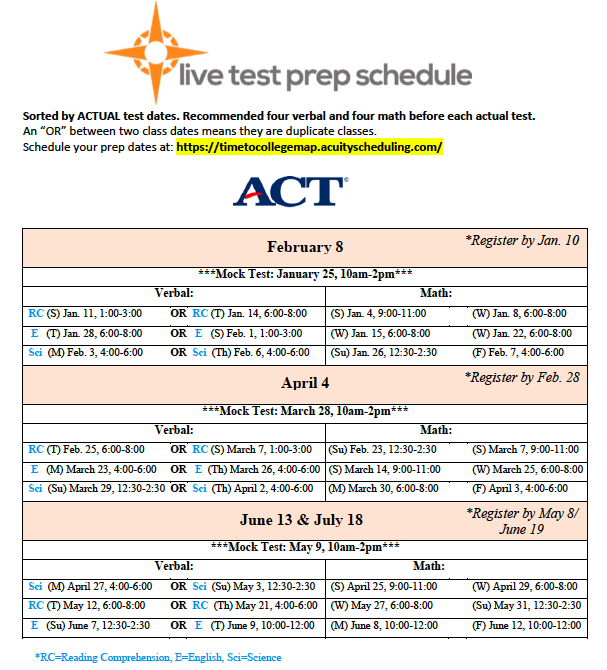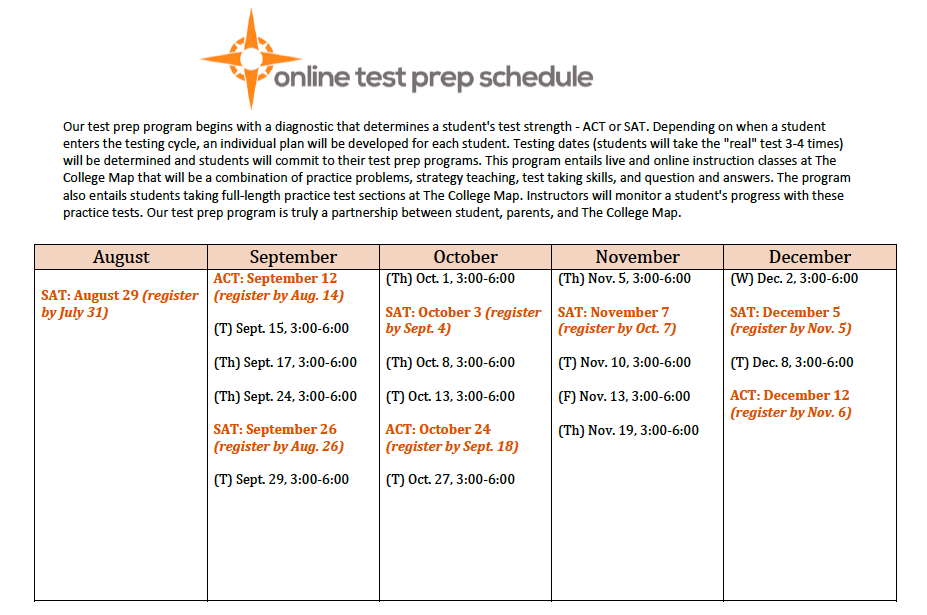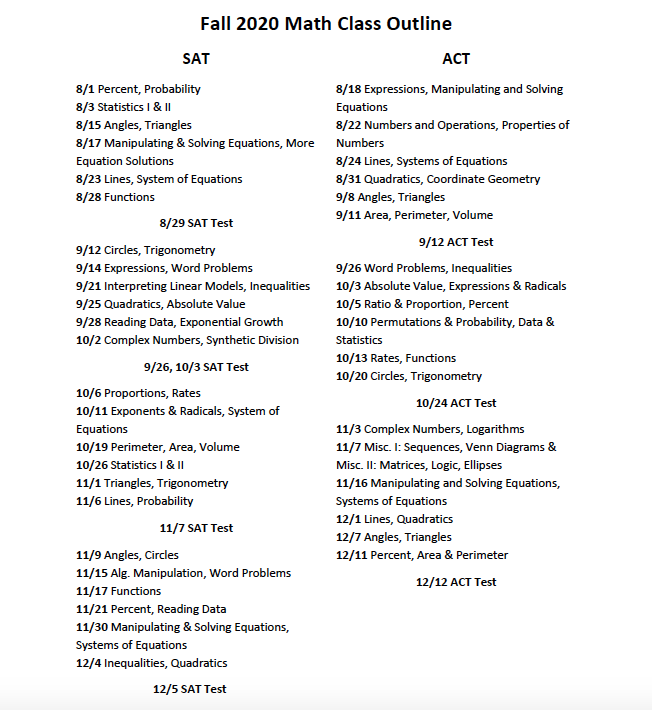table div table+table+table+table+table+table+table+table+table+table+table+table+table+table+table+table+table+table+table+table+table+table+table+table+table+table+table+table+table+table+table+table+table+table+table+table+table+table+table+table+table+table+table+table+table+table+table+table+table+table div table{width:100%;padding:0}table div table+table+table+table+table+table+table+table+table+table+table+table+table+table+table+table+table+table+table+table+table+table+table+table+table+table+table+table+table+table+table+table+table+table+table+table+table+table+table+table+table+table+table+table+table+table+table+table+table+table div table img{width:96.23%;padding:0;float:none}table div table+table+table+table+table+table+table+table+table+table+table+table+table+table+table+table+table+table+table+table+table+table+table+table+table+table+table+table+table+table+table+table+table+table+table+table+table+table+table+table+table+table+table+table+table+table+table+table+table+table div table td{width:100%;padding:0 1.88% 18px}/* styles */## SAT Subject Tests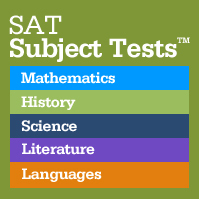/* styles */ Due to the cancelation of the May and June SAT exams, many students were not able to sit for their SAT Subject Tests. Please remember to re-schedule those exams for October 3rd! Subject Tests will not be offered in September. Subject tests are subject specific college admissions exams. They are designed by the same company (College Board) that publishes the SAT and AP exams. There are twenty different subject tests available in the areas of English, Math, Science, and foreign language. They are one hour long multiple choice tests that are given on the dates that the SAT is given- but, not all twenty tests are offered on the same date. Here is a list of updated colleges requiring SAT Subject Tests.
 table div table+table+table+table+table+table+table+table+table+table+table+table+table+table+table+table+table+table+table+table+table+table+table+table+table+table+table+table+table+table+table+table+table+table+table+table+table+table+table+table+table+table+table+table+table+table+table+table+table+table+table+table+table+table div table{width:100%;padding:0}table div table+table+table+table+table+table+table+table+table+table+table+table+table+table+table+table+table+table+table+table+table+table+table+table+table+table+table+table+table+table+table+table+table+table+table+table+table+table+table+table+table+table+table+table+table+table+table+table+table+table+table+table+table+table div table img{width:96.23%;padding:0;float:none}table div table+table+table+table+table+table+table+table+table+table+table+table+table+table+table+table+table+table+table+table+table+table+table+table+table+table+table+table+table+table+table+table+table+table+table+table+table+table+table+table+table+table+table+table+table+table+table+table+table+table+table+table+table+table div table td{width:100%;padding:0 1.88% 18px}/* styles */## Bright Futures/* styles */ Seniors, please make sure you submit a completed Florida Financial Aid Application (FFAA) in order to be considered for State Scholarship & Grant Programs. Seniors, please Create a Student Account. After logging into your account, you may proceed to complete the FFAA. Even IF you are not attending an in-state Florida school, we highly recommend every senior complete their Bright Futures application. Florida graduates have two years to enact their scholarship money. The FFAA opens on December 1st. The Bright Futures link can answer all of your questions:
 table div table+table+table+table+table+table+table+table+table+table+table+table+table+table+table+table+table+table+table+table+table+table+table+table+table+table+table+table+table+table+table+table+table+table+table+table+table+table+table+table+table+table+table+table+table+table+table+table+table+table+table+table+table+table+table+table+table+table div table{width:100%;padding:0}table div table+table+table+table+table+table+table+table+table+table+table+table+table+table+table+table+table+table+table+table+table+table+table+table+table+table+table+table+table+table+table+table+table+table+table+table+table+table+table+table+table+table+table+table+table+table+table+table+table+table+table+table+table+table+table+table+table+table div table img{width:96.23%;padding:0;float:none}table div table+table+table+table+table+table+table+table+table+table+table+table+table+table+table+table+table+table+table+table+table+table+table+table+table+table+table+table+table+table+table+table+table+table+table+table+table+table+table+table+table+table+table+table+table+table+table+table+table+table+table+table+table+table+table+table+table+table div table td{width:100%;padding:0 1.88% 18px}/* styles */## Monthly Scholarships/* styles */ There are thousands of scholarships out there. Below you will find many scholarships, most with general eligibility requirements. Click here for DoSomething.org Monthly Scholarships! Click here for Monthly Scholarships!
 table div table+table+table+table+table+table+table+table+table+table+table+table+table+table+table+table+table+table+table+table+table+table+table+table+table+table+table+table+table+table+table+table+table+table+table+table+table+table+table+table+table+table+table+table+table+table+table+table+table+table+table+table+table+table+table+table+table+table+table+table+table+table div table{width:100%;padding:0}table div table+table+table+table+table+table+table+table+table+table+table+table+table+table+table+table+table+table+table+table+table+table+table+table+table+table+table+table+table+table+table+table+table+table+table+table+table+table+table+table+table+table+table+table+table+table+table+table+table+table+table+table+table+table+table+table+table+table+table+table+table+table div table img{width:96.23%;padding:0;float:none}table div table+table+table+table+table+table+table+table+table+table+table+table+table+table+table+table+table+table+table+table+table+table+table+table+table+table+table+table+table+table+table+table+table+table+table+table+table+table+table+table+table+table+table+table+table+table+table+table+table+table+table+table+table+table+table+table+table+table+table+table+table+table div table td{width:100%;padding:0 1.88% 18px}/* styles */